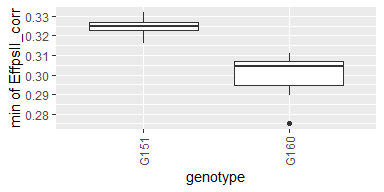# The statgenHTP Package

The statgenHTP package is developed as an easy-to-use package for analyzing data coming from high throughput phenotyping (HTP) platform experiments. The package provides many options for plotting and exporting the results of the analyses. It was developed within the EPPN2020project to meet the needs for automated analyses of HTP data.

New phenotyping techniques enable measuring traits at high throughput, with traits being measured at multiple time points for hundreds or thousands of plants. This requires automatic modeling of the data with a model that is robust, flexible and has easy selection steps.

The aim of this package is to provide a suit of functions to (1) detect outliers at the time point or at the plant levels, (2) accurately separate the genetic effects from the spatial effects at each time point, (3) model the temporal evolution of the genetic signal, and (4) estimate relevant parameters from a modeled time course. It will provide the user with either genotypic values or corrected values that can be used for further modeling, e.g. extract responses to environment .

Structure of the package

The overall structure of the package is in six main parts:

1. Data description and preparation - statgenHTP tutorial: 1. Introduction, data description and preparation
2. Outlier detection: single observations - statgenHTP tutorial: 2. Outlier detection for single observations
3. Correction for spatial trends - statgenHTP tutorial: 3. Correction for spatial trends
4. Outlier detection: series of observations - statgenHTP tutorial: 4. Outlier detection for series of observations
5. Modeling genetic signal - statgenHTP tutorial: 5. Modelling the temporal evolution of the genetic signal
6. Parameter estimation - statgenHTP tutorial: 6. Estimation of parameters for time courses

This document gives an overview of these steps and how to use the package to perform an analysis. For a more in depth description of the data, a theoretical background for the different steps and examples with additional data sets see the package website.

# Data description

## Example 1: photosystem efficiency in Arabidopsis

The used in this vignette contains data from an experiment in the Phenovator platform (WUR, Netherlands, ) with Arabidopsis plants. It consists of one experiment with 1440 plants grown in a growth chamber with different light intensity. The data set called “PhenovatorDat1” is included in the package.

The number of tested genotypes (Genotype) is 192 with 6-7 replicates per genotype (Replicate). Four reference genotypes were also tested with 15 or 30 replicates. The studied trait is the photosystem II efficiency (EffpsII) extracted from the pictures over time . The unique ID of the plant is recorded (pos), together with the pot position in row (x) and in column (y). The data set also includes factors from the design: the position of the camera (Image_pos) and the pots table (Basin).

data("PhenovatorDat1")
Genotype Basin Image_pos Replicate x y Sowing_Position timepoints EffpsII pos
G001 2 1b 8 14 32 8R02 2018-05-31 16:37:00 0.685 c14r32
G001 2 1b 8 14 32 8R02 2018-06-01 09:07:00 0.688 c14r32
G001 2 1b 8 14 32 8R02 2018-06-01 11:37:00 0.652 c14r32
G001 2 1b 8 14 32 8R02 2018-06-01 14:37:00 0.671 c14r32
G001 2 1b 8 14 32 8R02 2018-06-01 16:37:00 0.616 c14r32
G001 2 1b 8 14 32 8R02 2018-06-02 09:07:00 0.678 c14r32

# Data preparation

The first step when modeling platform experiment data with the statgenHTP package is creating an object of class TP (Time Points). In this object, the time points are split into single data.frames. It is then used throughout the statgenHTP package as input for analyses.

## Create a TP object containing the data from the Phenovator.
phenoTP <- createTimePoints(dat = PhenovatorDat1,
experimentName = "Phenovator",
genotype = "Genotype",
timePoint = "timepoints",
repId = "Replicate",
plotId = "pos",
rowNum = "y", colNum = "x",
checkGenotypes = c("check1", "check2", "check3", "check4"))
#> Warning: The following plotIds have observations for less than 50% of the time points:
#> c24r41, c7r18, c7r49
summary(phenoTP)
#> phenoTP contains data for experiment Phenovator.
#>
#> It contains 73 time points.
#> First time point: 2018-05-31 16:37:00
#> Last time point: 2018-06-18 16:37:00
#>
#> The following genotypes are defined as check genotypes: check1, check2, check3, check4.

In this data set, 3 plants contain less than 50% of the 73 time points. The user may choose to check the data for these plants and eventually to remove them from the data set.

The function getTimePoints() allows to generate a data.frame containing the time points and their numbers in the TP object. Below is an example with the first 6 time points of the phenoTP:

## Extract the time points table.
timepoint <- getTimePoints(phenoTP)
1 2018-05-31 16:37:00
2 2018-06-01 09:07:00
3 2018-06-01 11:37:00
4 2018-06-01 14:37:00
5 2018-06-01 16:37:00
6 2018-06-02 09:07:00

# Data visualization

Several plots can be made to further investigate the content of a TP object.

## Layout plot

The first type of plot displays the layout of the experiment as a grid using the row and column coordinates. The default option creates plots of all time points in the TP object. This can be restricted to a selection of time points using their number in the option timePoints. If repId was specified when creating the TP object, replicate blocks are delineated by a black line. Missing plots are indicated in white enclosed with a bold black line. This type of plot allows checking the design of the experiment.

## Plot the layout for the third time point.
plot(phenoTP,
plotType = "layout",
timePoints = 3)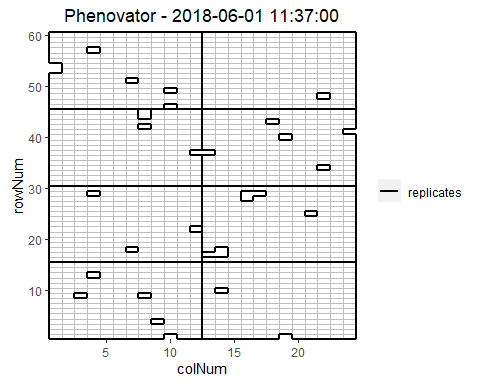Here, the third time point is displayed which corresponds to the 1st of June 2018 at 11:37. Note that the title can be manually changed using the title option. This plot can be extended by highlighting interesting genotypes in the layout. Hereafter the check genotypes are highlighted:

## Plot the layout for the third time point with the check genotypes highlighted.
plot(phenoTP,
plotType = "layout",
timePoints = 3,
highlight = c("check1", "check2", "check3", "check4"))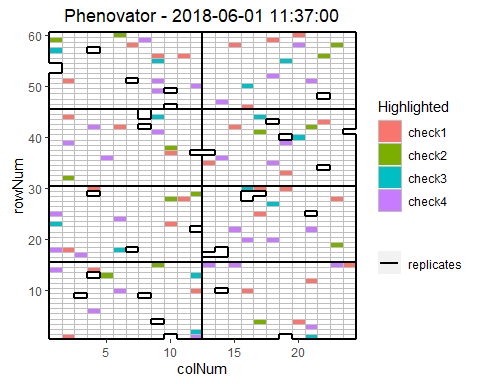It is possible to add the labels of the genotypes to the layout.

## Plot the layout for the third time point.
plot(phenoTP,
plotType = "layout",
timePoints = 3,
highlight = c("check1", "check2", "check3", "check4"),
showGeno = TRUE)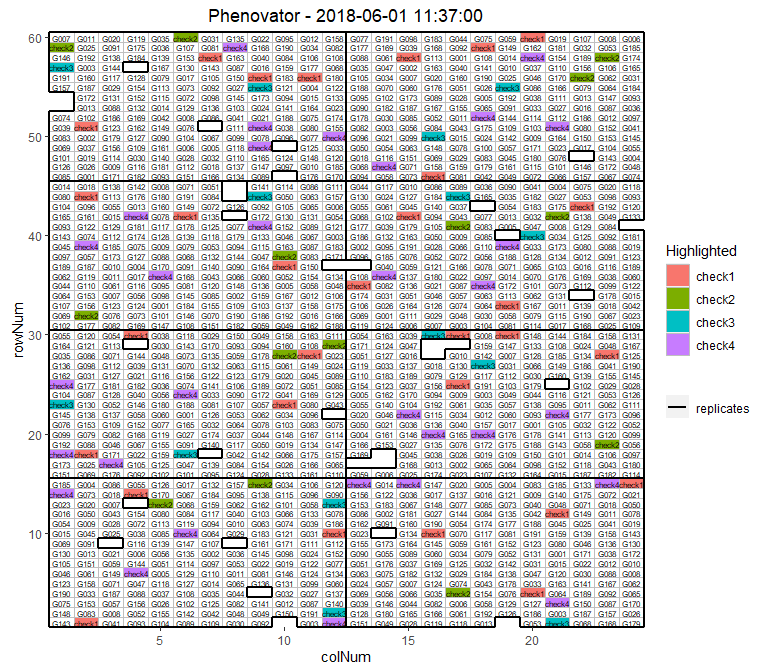We can visualize the raw data of a given trait on the layout, as a heatmap. This type of plot gives a first indication of the spatial variability at a given time point. This can be further investigated with the spatial modeling.

## Plot the layout for the third time point.
plot(phenoTP,
plotType = "layout",
timePoints = 3,
traits = "EffpsII")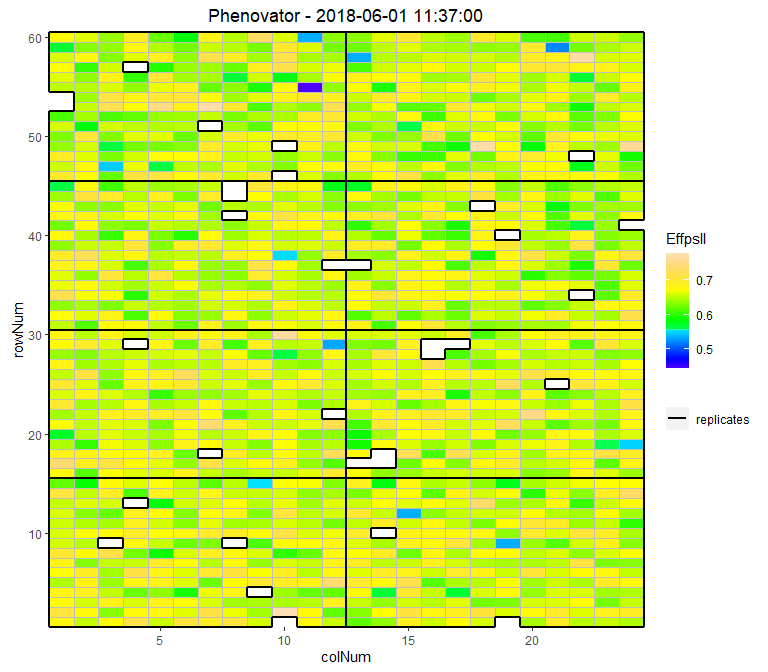## Raw data plot

Raw data can be displayed per genotype with one color per plotId.

## Create the raw data time courses for three genotypes.
plot(phenoTP,
traits = "EffpsII",
plotType = "raw",
genotypes = c("G001", "G002", "check1"))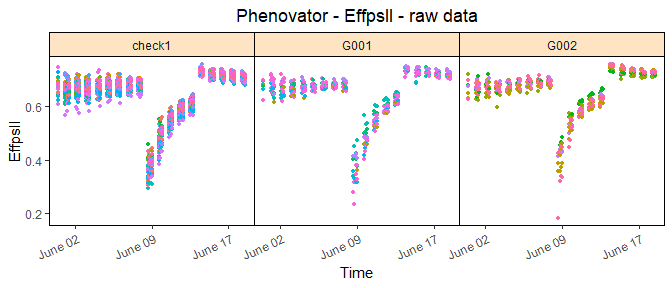## Boxplot

Boxplots can be made to visually assess the variability of the trait(s) in the TP object. By default a box is plotted per time point for the specified trait using all time points.

## Create a boxplot for "EffpsII" using the default all time points.
plot(phenoTP,
plotType = "box",
traits = "EffpsII")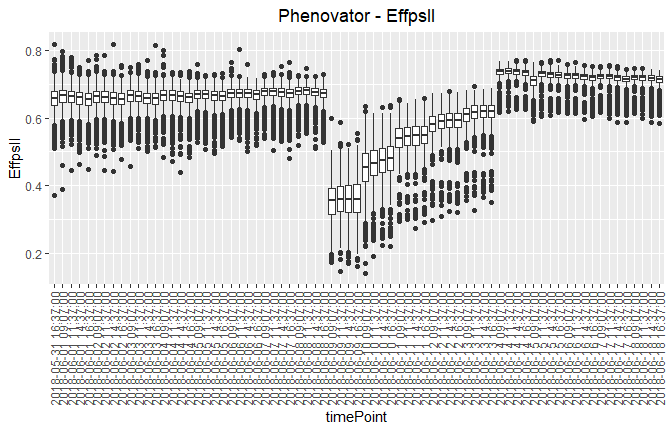## Correlation plot

Finally, a plot of the correlations between the observations in time for a specified trait can be made. The order of the plot is chronological and by default all time points are used.

## Create a correlation plot for "EffpsII" for a selection of time points.
plot(phenoTP,
plotType = "cor",
traits = "EffpsII",
timePoints = seq(from = 1, to = 73, by = 5))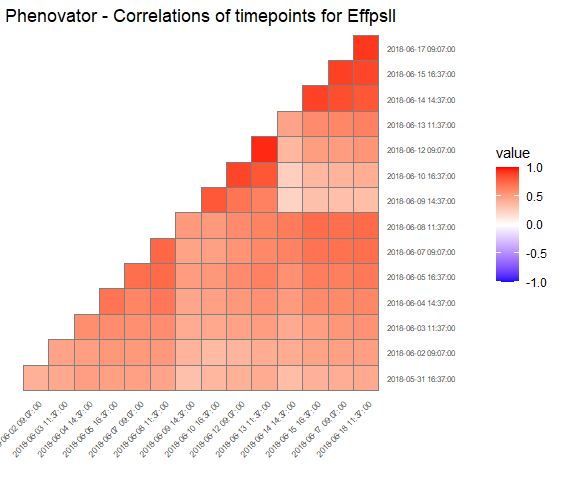# Outlier detection for single observations

Time courses of phenotypic data are viewed as continuous time-related functions. The first cleaning step consists of roughly checking the consistency of each point with its neighbors within a time course. Outlying single observations are measurements that do not follow the expected behavior at a given time. The detection of outlying observations is done “one time course after the other”.

Local regression is used to locally approximate the time courses by parametric functions. First a local regression is fitted at a set of points, then the fit is interpolated to other points. A confidence interval can then be calculated. Points outside this interval will be annotated as outliers.

# First select a subset of plants, for example here 4 plants.
plantSel <- c("c1r17","c13r17","c6r51","c21r24")
# Then run on the subset
resuVatorHTP <- detectSingleOut(TP = phenoTP,
trait = "EffpsII",
plotIds = plantSel,
confIntSize = 3,
nnLocfit = 0.1)
plotId timePoint EffpsII yPred sd_yPred lwr upr outlier
c13r17 2018-06-03 09:07:00 0.751 0.6852574 0.0389194 0.5684992 0.8020156 0
c13r17 2018-06-03 11:37:00 0.538 0.6833963 0.0255016 0.6068916 0.7599011 1
c13r17 2018-06-03 14:37:00 0.737 0.7045487 0.0342136 0.6019078 0.8071896 0
c13r17 2018-06-04 09:07:00 0.619 0.6330957 0.0436610 0.5021126 0.7640788 0
c13r17 2018-06-04 11:37:00 0.711 0.6655915 0.0322765 0.5687620 0.7624211 0
c13r17 2018-06-04 14:37:00 0.633 0.6861254 0.0330415 0.5870010 0.7852499 0

The predicted values and the confidence interval can be visualized using the plot function.

plot(resuVatorHTP,
outOnly = FALSE)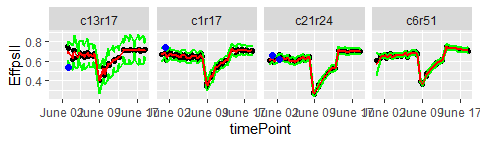The annotated points can be replaced by NA for the studied trait using the function removeSingleOut(). It creates a new TP object.

phenoTPOut <- removeSingleOut(phenoTP,
resuVatorHTP)

# Outlier detection for series of observations

After correcting the data for spatial trends, we can now check if the data contains outliers for series of observations. We do this by modeling each time course using a non-parametric smoothing spline with a fixed number of knots. The estimates for the spline coefficients are then extracted per time course (typically per plant) and correlations between those coefficient vectors are calculated to identify outlying time courses, i.e., plants.

The function fitSpline() fits a P-spline per plant for the selected trait. The function output contains the P-spline model coefficients and the values predicted on a dense grid using the P-spline model coefficients.

data(spatCorrectedVator)
# Fit P-splines using on a subset of genotypes.
subGenoVator <- c("G160", "G151")

fit.spline <- fitSpline(inDat = spatCorrectedVator,
trait = "EffpsII_corr",
genotypes = subGenoVator,
knots = 50,
# Extracting the tables of predicted values and P-spline coefficients
predDat <- fit.spline$predDat coefDat <- fit.spline$coefDat

We can then visualize the P-spline predictions and first derivatives for a subset of genotypes or for a subset of plots.

plot(fit.spline,
genotypes = "G160")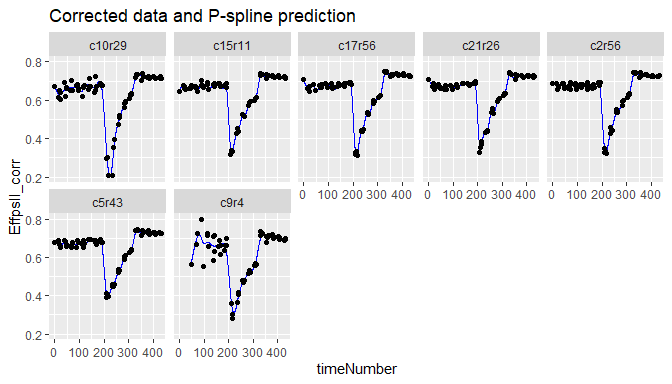The coefficients are then used to tag suspect time courses with the function detectSerieOut().

outVator <- detectSerieOut(corrDat = spatCorrectedVator,
predDat = predDat,
coefDat = coefDat,
trait = "EffpsII_corr",
genotypes = subGenoVator,
thrCor = 0.9,
thrPca = 30)
plotId genotype reason value
c21r25 G151 mean corr 0.7620638
c21r25 G151 angle 39.8776276
c9r4 G160 mean corr 0.6778041
c9r4 G160 angle 47.3176970
c9r4 G160 slope 0.6046173

Visualizing the results we see that for genotype G151 plant c21r25 is tagged as outlier:

plot(outVator, genotypes = "G151")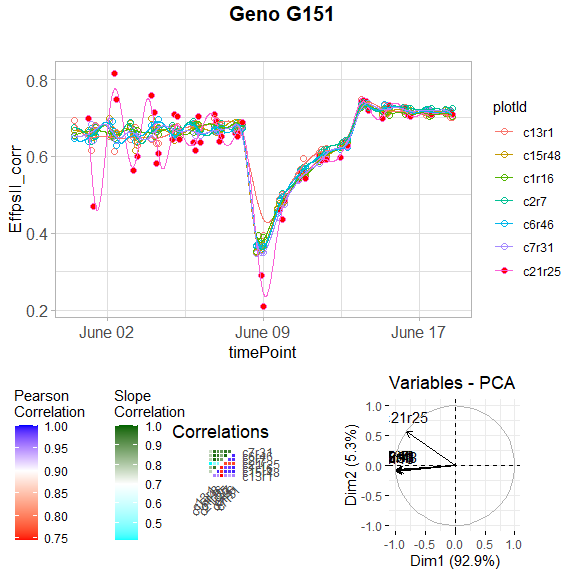We can then remove the outlying plants from the data set or fitted spline.

fit.splineOut <- removeSerieOut(fitSpline = fit.spline,
serieOut = outVator)

# Modeling the genetic signal

This section presents the second stage of the two-stage approach proposed by Pérez-Valencia et al. (2022). The aim is modeling the temporal evolution of the genetic signal after a spatial correction is performed on a phenotypic trait. Data consist of time-series (curves) of a (possibly) spatially corrected plant/plot phenotype. We assume that data present a hierarchical structure with plots nested in genotypes, and genotypes nested in “populations”. To model this sample of curves an additive decomposition of the phenotypic variation over time is considered, and a P-spline-based three-level nested hierarchical data model (hereafter refer as psHDM) is used.

To illustrate the analysis, we use the Arabidopsis data corrected for spatial trends, spatCorrectedVator. For simplicity, we will assume one population (pop) and two genotypes (genotypes in subGenoVator). The data structure and the new “population” variable are as follows

data(spatCorrectedVator)
spatCorrectedVator[["pop"]] <- as.factor(rep("Pop1", nrow(spatCorrectedVator)))
str(droplevels(spatCorrectedVator[spatCorrectedVator$genotype %in% subGenoVator,])) #> 'data.frame': 995 obs. of 14 variables: #>$ timeNumber  : int  1 1 1 1 1 1 1 1 1 1 ...
#>  $timePoint : POSIXct, format: "2018-05-31 16:37:00" "2018-05-31 16:37:00" ... #>$ EffpsII_corr: num  0.666 0.654 0.646 0.692 0.675 ...
#>  $EffpsII : num 0.663 0.655 0.655 0.706 0.672 0.649 0.692 0.67 0.714 0.674 ... #>$ wt          : num  36 36 36 36 36 ...
#>  $genotype : Factor w/ 2 levels "G151","G160": 1 1 1 1 1 1 2 2 2 2 ... #>$ check       : Factor w/ 1 level "noCheck": 1 1 1 1 1 1 1 1 1 1 ...
#>  $repId : Factor w/ 7 levels "1","2","3","4",..: 7 2 4 6 1 3 7 4 5 2 ... #>$ Image_pos   : Factor w/ 9 levels "1a","1b","1c",..: 3 1 7 8 5 4 2 2 7 8 ...
#>  $rowId : Factor w/ 13 levels "1","4","7","11",..: 12 5 11 1 3 9 13 13 7 8 ... #>$ colId       : Factor w/ 11 levels "1","2","5","6",..: 9 1 4 8 2 5 10 2 11 7 ...
#>  $plotId : Factor w/ 14 levels "c10r29","c13r1",..: 4 6 11 2 9 12 5 8 7 1 ... #>$ timeNumHour : num  0 0 0 0 0 0 0 0 0 0 ...

## Model prediction

The predict.psHDM() function can be used to obtain predictions from a fitted psHDM model (obtained using the fitSplineHDM() function; see above). In particular, this function allows obtaining predictions (estimated curves at each level of the hierarchy) on a dense grid of time points. Also, it allows the calculation of standard errors. These standard errors can be used to construct (approximate) pointwise confidence intervals for the estimated curves.

In the following code, we use the fit.psHDM object to make predictions at the three levels of the hierarchy (pred = list(pop = TRUE, geno = TRUE, plot = TRUE)), and to obtain standard errors at the population and genotype levels (se = list(pop = TRUE, geno = TRUE, plot = FALSE)). The original data is measured at $$73$$ time points, but predictions are obtained at $$100$$ time points in the same range than the original time points (argument newtimes).

## Predict P-spline HDM.
pred.psHDM <- predict(object = fit.psHDM,
newtimes = seq(min(fit.psHDM$time[["timeNumber"]]), max(fit.psHDM$time[["timeNumber"]]),
length.out = 100),
pred = list(pop = TRUE, geno = TRUE, plot = TRUE),
se = list(pop = TRUE, geno = TRUE, plot = FALSE),
trace = FALSE)

As result, three data frames with predictions (and standard errors) at population (pred.psHDM$popLevel), genotype (pred.psHDM$GenoLevel) and plot (pred.psHDM\$plotLevel) levels are returned.

## Model plot

The plot.psHDM() function plots psHDM objects. We note that objects of class psHDM can be obtained using both fitSplineHDM() and predict.psHDM() functions. In both cases, the resulting object contains information about estimated trajectories, deviations and first-order derivatives at the three levels of the hierarchy. As such, plots of these curves can be obtained. In addition, when plots are obtained from an object obtained using the predict.psHDM() function, $$95\%$$ pointwise confidence intervals are also depicted.

To illustrate the usage of function plot.psHDM(), we use here the object pred.psHDM obtained in the prediction section.

• If plotType = "popTra", the estimated population trajectory ($$\hat{f}_p(t)$$) is depicted and its $$95\%$$ pointwise confidence intervals. Additionally, the grey lines represent the observed trait that is used in the fitSplineHDM function.
plot(pred.psHDM, plotType = "popTra", themeSizeHDM = 10)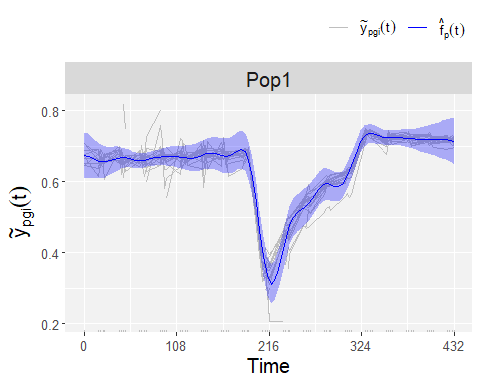• If plotType = "popGenoTra", estimated population ($$\hat{f}_p(t)$$) and genotype-especific ($$\hat{f}_p(t)+\hat{f}_{pg}(t)$$) trajectories are depicted for all genotypes. $$95\%$$ pointwise confidence intervals are depicted for the population trajectory. We let the user explore the plot for the first-order derivative of the estimated population and genotype trajectories (plotType = "popGenoDeriv").
plot(pred.psHDM, plotType = "popGenoTra", themeSizeHDM = 10)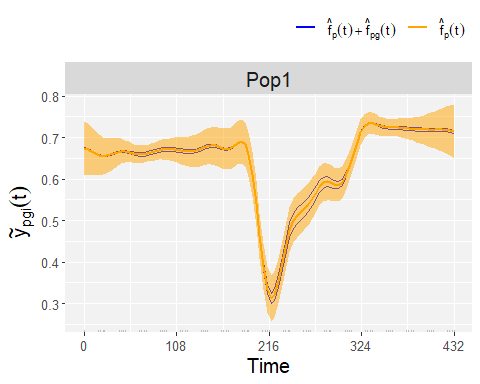• If plotType = "GenoDev", estimated genotype-specific deviations ($$\hat{f}_{pg}(t)$$) are depicted for all genotypes.
plot(pred.psHDM, plotType = "genoDev", themeSizeHDM = 10)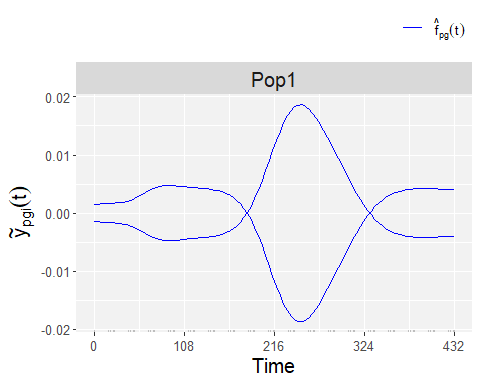Finally, if we are interested in obtaining plots at the plot level, we use plotType = "genoPlotTra". Here, estimated genotype ($$\hat{f}_p(t)+\hat{f}_{pg}(t)$$) and plot-specific ($$\hat{f}_p(t)+\hat{f}_{pg}(t)+\hat{f}_{pgi}(t)$$) trajectories are depicted for all plots separately for a selection of genotypes. Also, $$95\%$$ pointwise confidence intervals are depicted for the estimated genotype-specific trajectories.

plot(pred.psHDM,
plotType = "genoPlotTra",
themeSizeHDM = 10)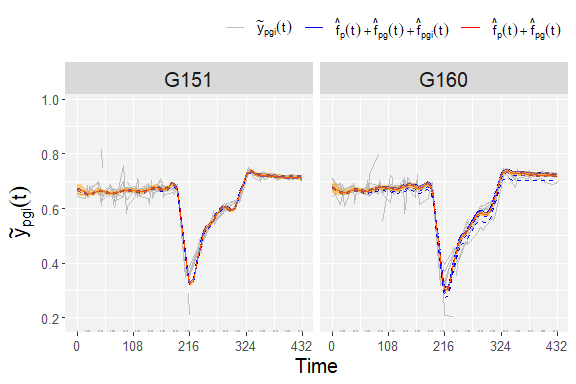# Estimation of parameters from time courses

The final step in the HTP data analysis is extracting interesting parameters from the modeled time courses. The parameters can then be further analyzed, for example in a GxE analysis (see statgenGxE), or a genetic analysis (see statgenGWAS).

paramVator1 <-
estimateSplineParameters(x = fit.splineOut,
estimate = "predictions",
what = "AUC",
timeMin = 330,
timeMax = 432,
genotypes = subGenoVator)

plot(paramVator1, plotType = "box")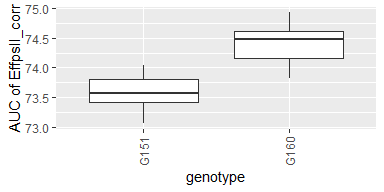These parameters can also be obtained from estimated time courses after the genetic signal is modeled with the psHDM model. For example, we can extract the minimum spatially corrected photosystem II efficiency (from previously estimated plot trajectories), for two genotypes (as illustration).

paramVator2 <-
estimateSplineParameters(x = pred.psHDM,
what = "min",
fitLevel = "plot",
estimate = "predictions")

plot(paramVator2, plotType = "box")